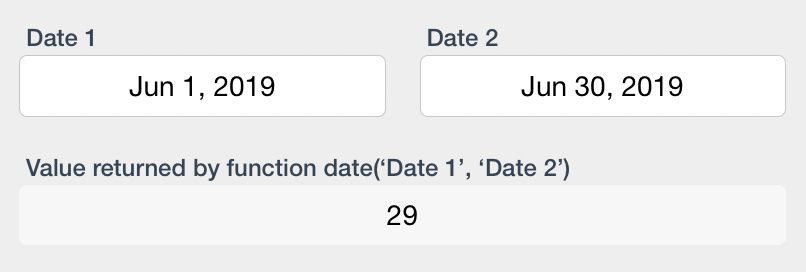### DESCRIPTION:

The days function is used to determine the number of days that will/have pass(ed) between the two dates that are the parameters to this function.

### SYNTAX

• days(‘Date Field 1’, ‘Date Field 2’)

WHERE

• days is the function keyword. This must be entered into the Ninox function editor in all lower-case characters
• ‘Date Field 1’ is a valid reference to a date field in the Ninox table schema
• ‘Date Field 2’ is a valid reference to a date field in the Ninox table schema

### Things to keep in mind when constructing this function:

• the days() function counts the number of days between two dates irrespective of whether or not a day occurs during the week (weekday) or on the weekend. To calculate the number of weekdays between two dates, refer to the workdays() function.

### EXAMPLE### USAGE

• The days() function is similar to the age() function in that it determines that amount of time (in days) that has passed between two dates. Where the age() function takes a single parameter and compares that parameter to the value of today(), the days() function takes two parameters thereby allowing the end user to determine the value of both the beginning and ending dates of the period.

Revision: 2